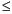Functions and CALL Routines

# COMB Function

Computes the number of combinations of n elements taken r at a time.
 Category: Combinatorial

## Syntax

 COMB(n, r)

### Arguments

n

is a nonnegative integer that represents the total number of elements from which the sample is chosen.

r

is a nonnegative integer that represents the number of chosen elements.

 Restriction: rn

The mathematical representation of the COMB function is given by the following equation:with n0, r0, and nr.

If the expression cannot be computed, a missing value is returned. For moderately large values, it is sometimes not possible to compute the COMB function.

SAS Statements Results
`x=comb(5,1);`
`5`

 Functions:Previous Page | Next Page | Top of Page